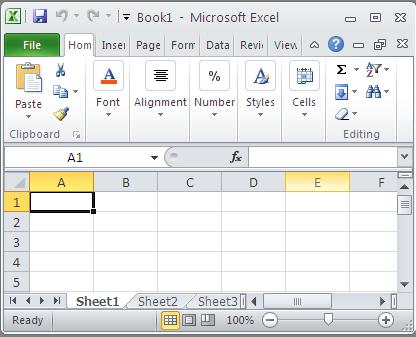#### What is Excel and Basic Knowledge You Need to Know

In this lesson, you get to know some basic definitions in Excel.Excel is a spreadsheet program that is part of the Microsoft Office suite of software. It is used to perform calculations, data analysis, and create charts. Excel is a powerful tool that can be used for a variety of purposes, including:

This article will teach you the basic knowledge you need to use Excel effectively. We will cover the following topics:

• What is Excel?
• Basic Excel terminology
• How to use Excel
• Things to remember
• Next steps

By the end of this article, you will have a good understanding of the basics of Excel and be able to use it to perform simple tasks.

## What is Excel?

Excel is a spreadsheet program that is used to store and organize data. It is a powerful tool that can be used for a variety of purposes, including:

• Performing calculations
• Creating charts and graphs
• Analyzing data
• Creating presentations

A spreadsheet is a grid of cells that can be used to store data. Each cell can contain text, numbers, formulas, or dates. Excel spreadsheets can be used to store large amounts of data and to perform complex calculations.

## Basic knowledge of Excel

Here are some basic Excel terminology that you need to know:

• Cell: A cell is the intersection of a row and column in a spreadsheet.
• Workbook: A workbook is a file that contains one or more worksheets.
• Worksheet: A worksheet is a single sheet of spreadsheet data.
• Formula: A formula is an expression that calculates a value based on the data in other cells.
• Function: A function is a pre-written formula that performs a specific task.
• Chart: A chart is a graphical representation of data.

## How to use Excel?

Excel is a complex program, but it is relatively easy to learn. The basic steps for using Excel are: